# What is momentum?

Momentum is a central concept in both classical and modern physics. It tells us something about the force that a massive body–or massless particle–exerts in a collision. But how are the classical and quantum formulas related? What exactly is momentum?

A textbook description of momentum merely characterizes the classical formula.

The formula gives a narrative in which momentum is a massive body in motion; but this description doesn’t represent the quantum mechanical formula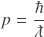in which radiation has no rest mass and velocity is fixed. For photons, momentum is derived from wavelength.

In order to give concrete meaning to the physical concept of momentum, we have to resolve the discrepancy between two distinct descriptions. Perhaps momentum encompasses separate ideas and we have a semantic inconsistency in how the word is used. Or, the two formulas may describe the same phenomenon in a way that is hidden by the equations. If we can explain how the two formulas describe the same physical phenomenon, then we can justify applying a single concept to both matter and radiation. In fact, we can do this in three simple steps.

## Step 1: Elementary formulas

A key to understanding relationships between classical and quantum formulas lies in the meaning of Planck’s constant. We can restate the reduced form of Planck’s constant ℏ in a more fundamental and physically meaningful way using Planck units:

It is mathematically equivalent to use Planck units as the values and unit dimensions match; but it is also evident that Planck units play a fundamental role in the equations of physics.

Next, we can restate three quantum formulas in terms of Planck units:

#### Momentum#### Compton wavelength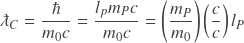#### de Broglie wavelength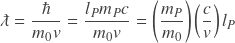We’ll use these three formulas to show certain relationships between classical and quantum mechanical properties.

## Step 2: Key relationships

The restated formulas above highlight two important relationships. The first is a relationship between a particle’s rest mass and its Compton wavelength. Ratios of each quantity to the Planck scale are equal:.

This equality can be stated in terms of rest mass as

(1)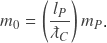The formulas show a second relationship between classical and quantum mechanical properties in that the ratio of Compton wavelength to de Broglie wavelength is equal to the ratio of velocity to the speed of light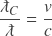.

This proportionality can be stated in terms of velocity

(2)These two formulas show how rest mass and velocity are related to the quantum mechanical property of wavelength. Note that we didn’t even need the elementary formulas to show the proportionality between wavelength and velocity, and the relationship between Compton wavelength and rest mass is well known. However, these relationships are easier to recognize in the more granular elementary equations.

## Step 3: Comparing quantum and classical formulas

Equations 1 and 2 give the following relationship between classical and quantum mechanical momentum formulas: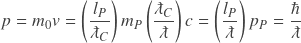.

In the classical formula, rest mass quantifies a particle’s Compton wavelength which is its minimum length as the particle approaches the speed of light (in non-relativistic terms). However, wavelength increases as velocity decreases in equal proportions; so the velocity term produces the particle’s de Broglie wavelength by applying the correct proportionality.

Momentum is therefore the same concept in classical and quantum systems. The physical meaning normally reserved for radiation also pertains to matter, and the meaning associated with the classical formula is misleading. Rest mass and velocity are bulk properties of matter that we use for calculating the energy potential of constituent matter particles–determined by their wavelengths. In this sense, the classical and quantum formulas are analogous to the relationship between a thermodynamic system and the individual particles comprising the system.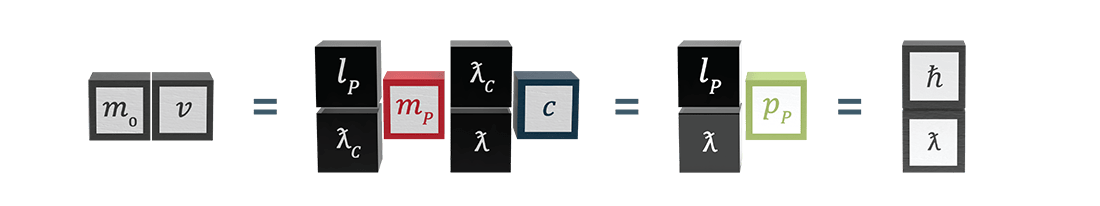Restating Planck’s constant in fundamental Planck units gives consistent physical meaning to classical and quantum mechanical momentum formulas.

It is easy to see that the classical and quantum mechanical formulas produce the same quantity of momentum when applying both formulas to a ground state electron:

#### Classical formula:

𝑝 = 𝑚𝑣 = (9.109 x 10-31 kg) (2,187,691 m/s) = 1.99 x 10-24 kgm/s

#### Quantum mechanical formula:= (1.055 x 10-34 kgm2/s) / (5.292 x 10-11 m) = 1.99 x 10-24 kgm/s

## The physical meaning of momentum

A consistent meaning arises for all particles when we treat momentum as a type of energy potential due strictly to wavelength and not velocity. The concept of momentum pre-dates quantum theory when Louise de Broglie first introduced wave-like attributes of matter. For Newton and others who described momentum prior to the 20th century, 𝑣2 simply matched the proportionality between rest mass and kinetic energy. Now that we understand the wave nature of matter, we can identify the distinct roles of wavelength and velocity in producing a squared quantity of kinetic energy. Not coincidentally, this explanation gives a single description of momentum for matter and radiation.

Momentum is a static property not represented by unit dimensions LT-1. Velocity is only a tool for obtaining particle wavelengths from a quantity of rest mass. Removing unit dimensions of velocity gives a quantity of effective mass in unit dimension M that is inversely proportional to an elementary particle’s wavelength. The simple formula for effective massworks for both matter and radiation, although it is pragmatic in many-particle systems to calculate using the bulk properties of rest mass and velocity. To restate effective mass in traditional unit dimensions of momentum, simply multiply by 𝑐.

So momentum is not a massive body in motion, but a quantity of energy potential inversely proportional to a particle’s wavelength. Energy is the attainment of that potential when velocity is considered. We can think of momentum as analogous to a payload–quantified by the concentration of a particle’s wavelength–while velocity delivers the payload as energy.

The insight needed to understand the physical meaning of momentum is that an equipartition of energy governs changes in a massive particle’s wavelength and velocity, making the two proportional. This relationship is easy to overlook unless one takes a critical look at the physical meaning of mathematical expressions.

### 1 thought on “What is momentum?”

1.